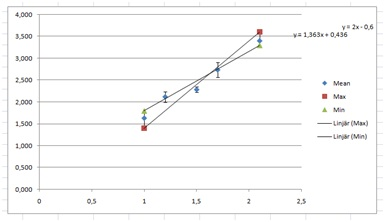# The Standard Error of the Mean, when does it work?

Up a level : IB Physics
Previous page : How to make a nice graph
Next page : How it is actually doneSEM – when does it work?

The standard error of he mean, SEM, is calculated as the standard deviation of our measurements for a given input, divided by the square root of the number of measurements.

As we have seen trough the previous pages this trick does sometimes work, and sometimes not. Why so?

Let us say we have an scale that is capable of measuring masses to within a  gram, and it shows the mass consistently to within a gram, then we cannot know the mass better than to within a gram however many measurements we do.  If the errors we see is due to the resolution of the instrument, then more measurements will not increase our knowledge of the position of the mean.

Say for example that you do 100 measurements, all of them giving you the value 17 grams. The standard deviation would be 0, and the SEM would be 0 too. Does that mean that you know the mass to be exactly 17 grams? No, certainly not.

In the cases where the instruments resolution is the main reason for errors in the measurements, the SEM- trick  would give us unreasonable small errors. We can see this in a graph when we find that we cannot find  lines of  the minimum and the maximum slopes that fits through the error bars, or that gives us unreasonable small errors. We can see an example of this below.If the error on the other hand is due to some random  source, say reaction times or random noise in an electric wire, then the SEM trick might work. I.e. when the underlying problem is not the accuracy of instrument but some other random source. This source could be within the instrument though. If the instrument shows values to within a gram, but actually randomly measures to within five grams, then the instrument has some internal randomness, say from electric noise, and then the SEM-trick might work.

If you look at your data, and you can see that the standard deviation is close to, or smaller than the resolution of the instrument, then it is usually best to use the resolution of the instrument as your error. I.e for a scale measuring to within a gram, use ±0.5 g.

On the other hand, if the SEM is bigger than or close to the resolution of the instrument, then try to use that at first. If the we cannot find  lines of  the minimum and the maximum slopes that fits through the error bars, then try using the standard deviation instead – and mention this in your work.Up a level : IB Physics
Previous page : How to make a nice graph
Next page : How it is actually doneLast modified: Jan 7, 2017 @ 17:28